# Angles 1

It is true neighboring angles have not common arm?

Result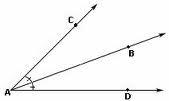We would be pleased if you find an error in the word problem, spelling mistakes, or inaccuracies and send it to us. Thank you!## Next similar math problems:

• Rhombus anglesIf one angle in the rhombus is 159°, what is it neighboring angle in rhombus?
• PoolIf water flows into the pool by two inlets, fill the whole for 19 hours. The first inlet filled pool 5 hour longer than the second. How long pool take to fill with two inlets separately?
• My fatherMy father has a big farm. 6/8 of it were planted with mango trees, 1/2 of the remainder are guava trees and the rest 10 trees are santol trees. What is the number of all trees?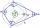What size have angles in quadrilateral (4-gon) if they are in a ratio of 8: 9: 10: 13?
• Four prismsQuestion No. 1: The prism has the dimensions a = 2.5 cm, b = 100 mm, c = 12 cm. What is its volume? a) 3000 cm2 b) 300 cm2 c) 3000 cm3 d) 300 cm3 Question No.2: The base of the prism is a rhombus with a side length of 30 cm and a height of 27 cm. The heig
• Railways - golden parachutes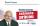As often happens in Slovakia habit, the state's financial institution which takes from poverty and gorilas give. A hardworking punishing taxes. Let's look at a short work of director Railway Company ZSSK - Mgr . P. K. : 18 months 'work' as director reduct
• Top of the towerThe top of the tower has the shape of a regular hexagonal pyramid. The base edge has a length of 1.2 m, the pyramid height is 1.6 m. How many square meters of sheet metal is needed to cover the top of the tower if 15% extra sheet metal is needed for joint
• Tennis aces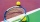The number of aces served by Novak Djokovic in the last 20 tournaments that he has participated in, is shown below. 12 17 13 7 8 14 11 14 10 12 15 9 11 13 6 15 18 5 19 24 1.1 using the raw, determine the range. 1.2 Group the data into a frequency distribu
• AnglesThe outer angle of the triangle ABC at the vertex A is 114°12'. The outer angle at the vertex B is 139°18'. What size is the internal angle at the vertex C?
• n-gon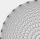Gabo draw n-gon, which angles are consecutive members of an arithmetic sequence. The smallest angle is 70° biggest 170°. How many sides have Gabo's n-gon?
• Complementary angles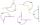Two angles are complementary. If one angle measures 10°, what is the measure of the second angle?
• Tablecloth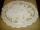I embroider tablecloth in 20 days but if I embroider 3/4 hours a day I have embroider it for 15 days. How long do I embroider a day?
• Angles ratioIn a triangle ABC true relationship c is less than b and b is less than a. Internal angles of the triangle are in the ratio 5:4:9. The size of the internal angle beta is:
• Pumps 3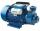Two pumps of the same power fill the garden pool for 10 hours. How many of these pumps would have to use if we want to shorten the filling of the pool to four hours?
• Janitor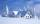Janitor tosses 26 cm layer of snow on the school grounds for 90 minutes. How many friends would have to call for help if snow needs to have bowled for 15 minutes. We assume that at the same time bow all the same amount of snow.
• TrianglesFind out whether given sizes of the angles can be interior angles of a triangle: a) 23°10',84°30',72°20' b) 90°,41°33',48°37' c) 14°51',90°,75°49' d) 58°58',59°59',60°3'
• PexesoDrawing paper has dimensions of 60cm and 840mm. Pupils have to divide it into squares so that they can make a pexeso. What dimension must squares have if their side is to be larger than 3cm and less than 10cm?